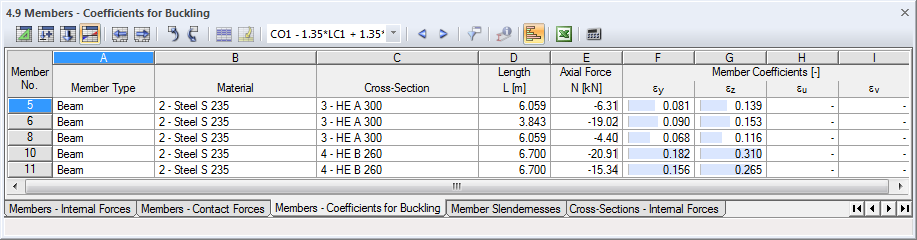# RFEM – Online Manual

Online manuals, introductory examples, tutorials, and other documentation.

# 8.9 Members - Coefficients for Buckling

When you calculate member models subjected to pressure according to the second-order analysis, the member coefficient ε is important (see Chapter 7.3.1). Each member has its own member coefficient that is determined from the compressive force, member length, and member stiffness.

If necessary, members with member coefficients higher than 1 have to be analyzed according to the second-order analysis. Standards of some countries such as the USA also have rules where member coefficients must be limited.

Table 4.9 shows the member coefficients that are governing for buckling. There is no graphical output option.Figure 8.29 Table 4.9 Members - Coefficients for Buckling

The member coefficients are listed by member numbers.

Member Type

The member types are indicated for informational purposes (see Chapter 4.17). RFEM only determines member coefficients for members that are able to absorb compressive forces.

Material

Characteristics of the material affect the member stiffness.

Cross-Section

The cross-section's moments of inertia are required to determine the member stiffnesses.

Length L

The member lengths are indicated in table column D.

Axial Force N

The column lists the axial forces used for determining the member coefficient. These are the axial forces available in the member center (x = L/2).

Member coefficients are only determined for members that have compression forces in at least one portion of the member (truss girder) or along the entire member (compression member, buckling member, etc.).

Member Coefficients εy / εz

The member coefficient ε depends on the member length L, compressive force N, and stiffness E ⋅ I.

Table columns F and G show the member coefficients that refer to the local member axis system y or z. When asymmetrical cross-sections such as angles are used, two additional columns appear where member coefficients are also shown in relation to the principal axes u and v.